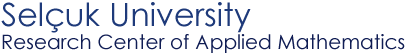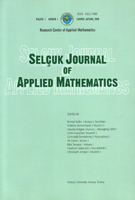Selçuk Journal of Applied Mathematics www.selcuk.edu.trSelçuk Journal of
Applied Mathematics

Winter-Spring, 2001
Volume  2
Number 1

Research Center of
Applied Mathematics

 SJAM Winter-Spring 2001, Volume 2 - Number 1 Hierarchical Cubature Formulas Vladimir Vaskevich Sobolev Institute of Mathematics, SB RAS, Novosibirsk, Russia email : vask@math.nsc.ru Received: March 10, 2001 Summary We study the properties of hierarchical bases in the space of continuous functions with bounded domain and construct the hierarchical cubature formulas. Hierarchical systems of functions are similar to the well-known Faber-Schauder basis. It is shown that arbitrary hierarchical basis generates a scale of Hilbert subspaces in the space of continuous functions. The scale in many respects is similar to the usual classification of functional spaces with respect to moothness. By integration over initial domain the standard interpolation formula for the given continuous integrand, we construct the hierarchical cubature formulas and prove that each of these formulas is optimal simultaneously in all Hilbert subspaces associated with the initial hierarchical basis. Hence, we have constructed the universally optimal cubature formulas. Key words calculation of integrals, guaranteed accuracy, cubature formulas, bases in Sobolev spaces, hierarchical bases Mathematics Subject Classification (1991): 65D32, 65D30, 41A55 Article in PS format (125 kb) Article in ZIP format (48 kb)
 The article is available to read in  Ghostview format
 [Home] [contact]